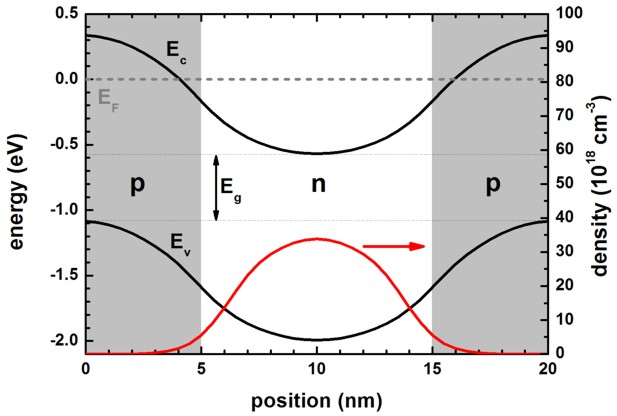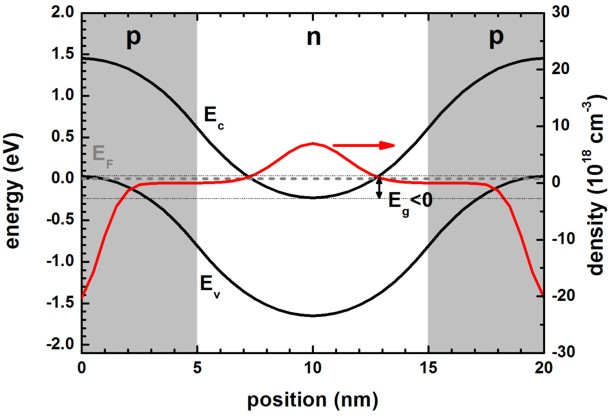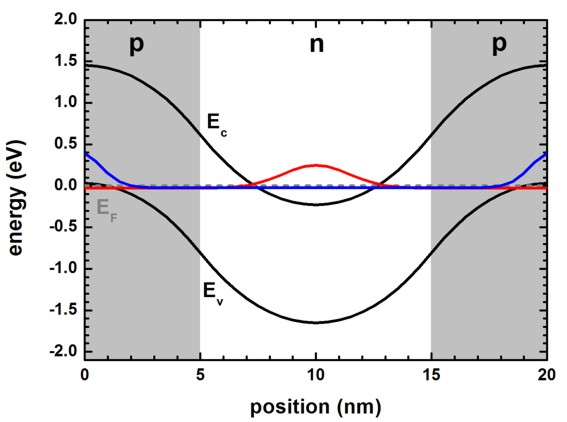nextnano.com  GUI: nextnanomat  Tool: nextnano++  Tool: nextnano³  Tool: nextnano.QCL  Download | Search | Copyright | News | Publications  * password protected nextnano³ software1D full-band density SL

# nextnano3 - Tutorial

## Density in np-doped GaAs superlattice - Comparison of classical, quantum, k.p and full-band density k.p approach for normal and broken gap alignment

Author: Stefan Birner
Please send comments to` support `(-at-)` nextnano.com`.

If you want to obtain the input file that is used within this tutorial, please contact stefan.birner@nextnano.de.
```a) ==> Density_GaAs_np_doped1D_cl.in                       - ```classical density``` ==> Density_GaAs_np_doped1D_qm.in                       - ```quantum density (single-band Schrödinger)``` ==> Density_GaAs_np_doped1D_qm_kp.in                    - ```quantum density (8-band k.p Schrödinger)``` ==> Density_GaAs_np_doped1D_qm_kp_fullband.in           - ```quantum density (8-band k.p Schrödinger full-band-density for electrons)``` ==> Density_GaAs_np_doped1D_qm_kp_fullband_hl.in        - ```quantum density (8-band k.p Schrödinger full-band-density for holes)``` b) ==> Density_GaAs_np_doped1D_broken_cl.in                - ```classical density``` ==> Density_GaAs_np_doped1D_broken_qm.in                - ```quantum density (single-band Schrödinger)``` ==> Density_GaAs_np_doped1D_broken_qm_kp.in             - ```quantum density (8-band k.p Schrödinger)``` - ```not possible !!!``` ==> Density_GaAs_np_doped1D_broken_qm_kp_fullband.in    - ```quantum density (8-band k.p Schrödinger full-band-density for electrons)``` ==> Density_GaAs_np_doped1D_broken_qm_kp_fullband_hl.in - ```quantum density (8-band k.p Schrödinger full-band-density for holes)```  ```

## Density in np-doped GaAs superlattice - Comparison of classical, quantum, k.p and full-band density k.p approach for normal and broken gap alignment

The aim of this tutorial is to compare the density calculation of different methods that are implemented in the nextnano³ software with respect to a broken gap situation.
It is a self-consistent Schrödinger-Poisson calculation.

This tutorial is similar to the discussion in

Self-consistent electronic structure method for broken-gap superlattices
T. Andlauer, T. Zibold, P. Vogl
Proc. SPIE 7222, 722211 (2009)

As an example, we use an np-doped bulk GaAs superlattice of periodic length 20 nm with periodic boundary conditions for the Schrödinger and Poisson equations.

• The temperature is set to 300 K.
• n-type region: 5 nm - 15 nm
• p-type region: 0 nm - 5 nm and 15 nm to 20 nm
•                                              n-type: The donor     concentration is 5.2 x 1019 cm`-`3 (fully ionized).
• a)       normal band alignment: p-type: The acceptor concentration is 2.6 x 1019 cm`-`3 (fully ionized)
b) broken gap band alignment: p-type: The acceptor concentration is 5.2 x 1019 cm`-`3 (fully ionized)
• In order to compare the 8-band k.p results to the simpler models for the density, we assume for all k.p calculations a parabolic and isotropic energy dispersion E(k) of electrons and holes where electrons and holes are decoupled. To achieve this, we use the following parameters:
EP = 0 eV
S = 1/me = 1/0.067
L = M = `-`3, N = 0 (or equivalently: gamma1 = 2, gamma2 = gamma3 = 0)
mhh = mlh = mso = 0.5 m0
• The number of grid points is 41, leading to a grid spacing of 0.5 nm.

a) normal band alignment, positive band gap Eg > 0, Ec > Ev

The following figure shows the self-consistently calculated conduction band edge Ec and highest valence band edge Ev for the normal band alignment, i.e. positive band gap Eg.
EF is the Fermi level. The p-type doped regions are indicated in grey, the n-type region in white.
These results are identical for single-band calculation, 8-band k.p calculation and full-band k.p calculation for electrons.
For full-band k.p calculation for holes, the only difference is that the electron density is zero, and that the hole density contains a negative charge density which is equal to the shown electron density, apart from the sign.
The classical calculation differs slightly. This is expected, since the structure represents a quantum well like structure exhibiting confinement and wave function penetration into the barrier.The electron density is contained in this file:``` densities/density1Del.dat ```The hole       density is contained in this file:``` densities/density1Dhl.dat  ```(for full-band density for holes)

b) broken band alignment, negative band gap Eg < 0, Ec < Ev

The following figure shows the results of the full-band k.p calculation where the band gap Eg of conduction to valence band edge alignment is negative.
The electron density is shown with positive sign (although it is of course negative in reality) so that this figure can be easier compared to the above one for normal band gap alignment.
If the density is negative as in the p-type region, it corresponds to hole density (which is of course positive in reality).The following figure shows the energy dispersion E(k||).In this figure, one cannot classify the energy states into pure electron and hole states as at certain wavevectors also mixed states occur.
In fact, this is not really true for this example.
In this example, we set the Kane parameter EP to zero, i.e. we decoupled electron states from hole states.
In this case we cannot get mixed states. We can only get electron states and hole states that have similar energy.

This is shown in the next figure which shows the square of the electron wave function (electron eigenvalue number 243 and 244, two-fold spin-degenerate),
and the square of the hole wave functions ("electron" eigenvalue number 245, 246, 247, 248, two-fold spin-degenerate, and light hole / heavy hole degenerate due to choice of k.p parameters)
for k|| number 9. The kx and ky values for this k|| number can be obtained from this file.
```Schroedinger_kp/kp_8x8k_parallel_qc001_el1D_per.dat k_par_number  k_x[1/Angstrom]  k_y[1/Angstrom] ... 9             0.0181818        0.0272727 k = (kx2 + ky2)1/2 = 0.0328 [1/Angstrom] = 0.328 [1/nm] ```The electron eigenvalue is below the "hole" eigenvalue energy in this figure.
For higher k|| vectors, this electron eigenvalue energy will be above the hole energy eigenvalue.In the full-band density k.p approach, both the red and the blue eigenstate will be occupied as an electron state.
By subtracting a positive background charge density, one gets the final density distribution.

## Full-band-density (8-band k.p)

The following switch is required to turn on "full-band density".

```\$numeric-control  ...  broken-gap = full-band-density```

As the structure consists of 41 grid points, we get 8 x 41 = 328 eigenstates for 8-band k.p in total.

Normal band gap situation:
The lowest 6 x 41 = 246 eigenstates belong to the hole states with their energies below the valence band edge.
There are 2 x 41 = 82 electron states above the conduction band edge.

• full-band density for electrons:``` \$quantum-model-electrons```
Here, we calculate all hole states, and the relevant electron states (```number-of-eigenvalues-per-band = 40```),
i.e. we need the eigenstate numbers 1 - 286, where 286 = 246 + ` 40`. For the output, we plot only``` 235 - 278```, i.e. the highest 12 holes states are included in the output of the wave functions.

`cb-num-ev-min = 235 ! `lower boundary for range of conduction band eigenvalues ``` cb-num-ev-max = 278 ! ```upper boundary``` ```For full-band density electrons, the eigenvalues are numbered from the bottom of the spectrum, with eigenvalue number 1 having the lowest energy, and being a hole eigenstate.
All eigenstates are treated as electrons, and occupied as electrons, and contribute to the (negative) electron charge density.
We then subtract a positive background charge density to obtain the final net charge density.
The file``` densities/density1Del.dat ```contains the electron charge carrier density which is positive in this example because a net electron density is present.```  ```
• full-band density for holes``` \$quantum-model-holes```
Here, we calculate all electron states, and the relevant hole states (```number-of-eigenvalues-per-band = 40  40  40```),
i.e. we need the eigenstate numbers 1 - 122, where 122 = 82 + ` 40`. For the output, we plot only``` 77 - 100```, i.e. the 6 lowest electron states are included in the output of the wave functions.

`vb-num-ev-min =  77 ! `lower boundary for range of valence band eigenvalues ``` vb-num-ev-max = 100 ! ```upper boundary``` ```For full-band density holes, the eigenvalues are numbered from the top of the spectrum, with eigenvalue number 1 having the highest energy, and being an electron eigenstate.``` ```All eigenstates are treated as holes, and occupied as holes, and contribute to the (positive) hole charge density.
We then subtract a negative background charge density to obtain the final net charge density.
The file``` densities/density1Dhl.dat ```contains the hole charge carrier density which is negative in this example because a net electron density is present.

Full-band-density holes (`\$quantum-model-holes`) is recommended, as one has less eigenvalues to calculate. This will make the numerical effort smaller.

• Please help us to improve our tutorial! Send comments to``` support [at] nextnano.com```.